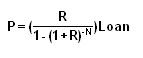# Fixed Rate Payment

Posted in Finance, Accounting and Economics Terms, Total Reads: 795

## Definition: Fixed Rate Payment

A Fixed rate payment is the kind of loan where the interest rate is fixed for the entire repayment period. This allows the lender and borrower to exactly calculate the future payments. Some of the other loans provided are floating in nature i.e. the interest rate is benchmarked on other rates such as the LIBOR + 2% rate. Thus when the LIBOR rate changes the interest payment changes simultaneously.

Fixed rate payment can also be defined as the constant amount paid by borrower to the lender in a period. If the repayment cycle is repeated at the end of every period, it is termed as ordinary annuity and if the repayment is to be done at the beginning of each term ,it is termed as annuity due. The loan is redeemed in equal instalments which covers both the principal and interest amount. The formulae to calculate the equal instalments is :The terms are defined as follows:

P is the amount paid for each term

R is the interest rate for the period

N is the number of periods

Loan is the total loan amount

R is the nominal interest rate for the period. If the interest charged is 12% annually and the payment period is taken to be monthly, then R for the period will be 12% / 12 = 1%.

Similarly N i.e. the number of period can be calculated by multiplying the number of years to the number of periods in a year. If the payment is done on quarterly basis and the number of years to repay the loan is 10 years then N= 4*10 i.e. 40 periods. Similarly if the repayment to be done is monthly in nature then N would be 120.

Hence, this concludes the definition of Fixed Rate Payment along with its overview.

Browse the definition and meaning of more terms similar to Fixed Rate Payment. The Management Dictionary covers over 7000 business concepts from 6 categories.

Search & Explore : Management Dictionary

Similar Definitions from same Category: